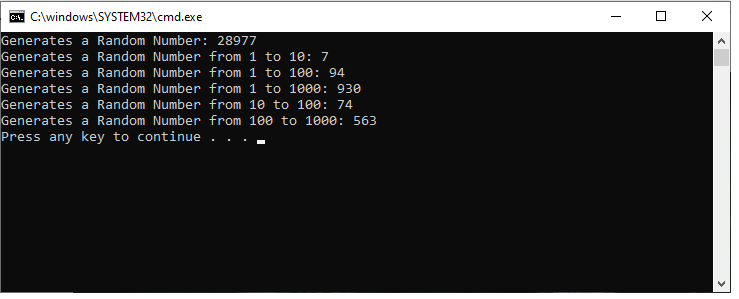﻿ How to Generate a Random Number in C++ ﻿# How to Generate a Random Number in C++In this article, we show how to generate a random number in C++.

In this case, we are specifically talking about integers.

We can generate a random number from any range such as any number from 1-10, or from 1-100, or from 1-1000.

To do this, we use the time and stdlib libraries.

The stdlib library contain the srand and rand functions.

The srand function allows us to initialize a random number generator.

The rand function allows us to generate a random number, in which we can input a function that returns a random number between a certain range specified.

So let's now go over the code, which is shown below.

The first thing we need to do is include all of the C++ libraries that our program needs.

We need the iostream to output the random numbers to the screen.

We need the stdlib library because it contains the srand and rand functions.

We need the time library because it is used to initialize the randon number generator.

So we have our main() function.

The line, srand(time(NULL));, sets the seed of the random number generator. This uses the computer's internal clock to select the seed. Since the time is always changing, this ensures that the seed is always changing. If it were to remain the same, the same random numbers would be generated each time the program is run.

We create a int variable, randomnumber, which we set equal to rand(). This is the simplest of all the random numbers we generate. This can generate any number from 0 to 32767. 32767 is the maximum random number from the rand function, which is RAND_MAX

Next, we create another random number, randomnumber10. This generates a number from 1 to 10. Since it goes to 10, we use, rand() % 10. Since we want it to go from 1 to 10, we use, +1. If we didn't do + 1, it would start at 0, not 1.

We then create another int variable, randomnumber100, which goes from 1 to 100.

We then create another int variable, randomnumber1000, which goes from 1 to 1000.

We then have generate random numbers that have different starting points other than 1.

We create an int variable, randomnumber_range10_100, which is set equal to, rand() % 100 + 10;

This generates a random number that goes from 10 to 100.

It starts at 10 because we have + 10.

We then create another int variable, randomnumber_range100_1000, which is set equal to, rand() % 1000 + 100;

It starts at 100 because we have + 100.

In this manner, you can generate a random integer from any range of integers in C++.

The output of this program is shown below.And this is how to generate a random number in C++.

Related Resources

How to Write to a File in C++